# Chapter 3.1 Simple Conditions

This chapter will discuss conditional statements in the Java language. Depending on the condition, a program may have different behavior. First will be explained the syntax of conditional operators if and if-else with appropriate examples. Then we will see in what range (scope) a variable lives. Finally, we will look through debugging techniques to track the step-by-step execution of our programs.

## Video

Watch the video lesson about what we will learn in this chapter: https://www.youtube.com/watch?v=cXKIVmjEgHw.

## Comparing numbers

In programming, we can compare values using the following operators:

• Operator < (less than)
• Operator > (greater than)
• Operator <= (less than or equals)
• Operator >= (greater than or equals)
• Operator == (equas)
• Operator != (not equals)

When comparing values, the result is of boolean type with a value true or false, depending on whether the result of the comparison is true or false.

### Examples for comparing numbers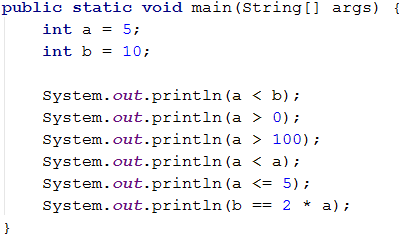### Comparison operators

In Java, we can use the following comparison operators when comparing numbers:

Operator description Notation
Equals to ==
Not Equals to !=
Greater than >
Gerater than or equals >=
Less than <
Less than or equals <=

The following example demonstrates how to use comparison operators in expressions:## Simple If conditions

In programming, we often check given conditions and perform different actions depending on the result. This is done by if conditional statement, which has the following structure:

if (condition) {
// body of if conditional statement
// single command or block of code to be executed if the condition is true
}


Read the grade from the console and check if it is excellent (≥ 5.50).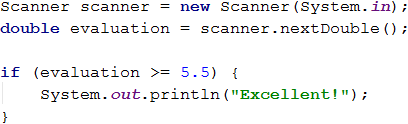Test the code (from the example) locally. Test with different grades, like 4.75, 5.49, 5.50, and 6.00. If the grade is less than 5.50, the program will not output any result, otherwise (if the grade is greater than or equals 5.50), the program will output "Excellent!".

## If-Else conditions

Simple if conditions could be extended with else conditional statement, which specifies a block of code to be executed, if the boolean expression (defined at the beginning if(condition)) returns false. The resulting conditional statement is called if-else construction and have the following behavior: if the condition returns positive (true) result – the code described in the curly brackets right after the if clause will be executed, otherwise if the condition returns negative (false) result – the code described in the curly brackets after the else clause will be executed. The format of the construction is:

if (condition) {
// body of if construction
// single command or block of code to be executed if the condition is true
} else {
// body of else construction
// single command or block of code to be executed if the condition is false
}


### Example: Excellent grade or not

Like the example above, read the grade from the console and check if it is excellent, but we should return the output in both cases.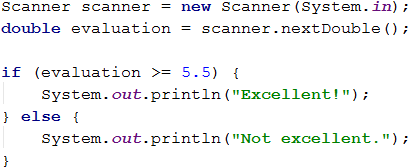## The curly brackets {} after If / Else

When we have only one command in the body of the if statement, we can skip the curly brackets. When we want to execute a block of code (group of commands), curly brackets are required, because if we skip them, only the first line after the if clause will be executed.It is a good practice to always put curly brackets, because it makes our code more readable and cleaner.

Here is an example that skipping curly brackets leads to confusion:Executing the above code will output the following result on the console:Here is the same example, but using curly brackets:Executing the code with curly brackets will output the following result on the console: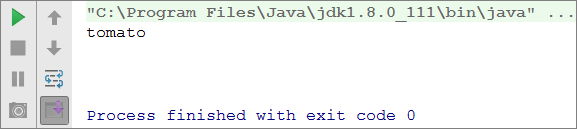Both use cases are correct and could be used depending on the situation and/or the specific requirements, but you always must be careful and check the expected results.

### Example: Even or odd number

Write a program that checks whether an integer is even or odd.

### Hint and guidelines

We can solve the problem with one if-else statement and the operator %, which returns the remainder of dividing two numbers.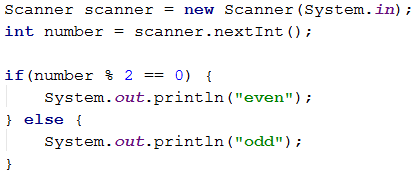Executing the above code will output the following result: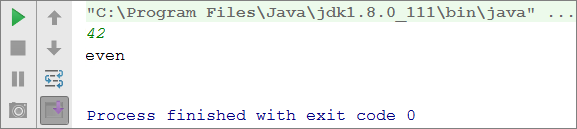### Example: The greater number

Write a program that reads two integer numbers, from the console, and return the greater one. Print the output in the following format: “Greater number: x”, where the x is the returned number.

### Hint and guidelines

Our first task is to read both integer numbers from the console. Then we must perform the check using one if-else statement in combination with the operator for greater than (>). Part of the code is consciously blurred to test what you have learned so far.Executing the above code will output the following result for numbers 3 and 5: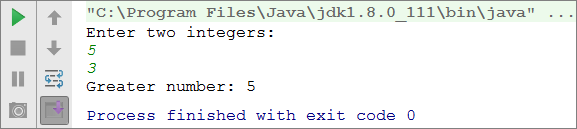## Variable scope

Each variable has a scope in which it exists, called variable scope. This scope determines where the variable lives, in other words the scope where you can use it. In Java, a variable scope begins from the line in which we defined it and ends with the first closing curly bracket } (of the method, of the if statement, etc.). Thus, it is important to know that any variable defined inside the body of an if statement will not be available outside of it, unless we have defined it above in the code.

In the example below we will get an error, because on the last line we are trying to print the variable salary that is defined inside the if statement, and we do not have access to it outside the block (in this case we will receive notification from the IDE about variable scope).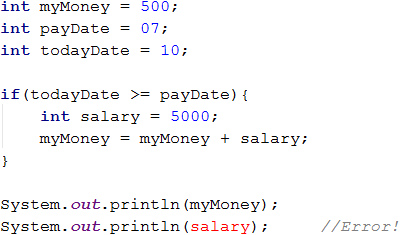## Sequence of If-Else conditions

Sometimes we need to do a sequence of conditions before we decide what actions our program will execute. In such cases, we can apply the construction if-else if… -else. For this purpose, we use the following format:

if (condition) {
// body of if statement
} else if (condition2) {
// body of if statement
} else if (condition3) {
// body of if statement
} … else {
// body of else statement
}


### Example: Digits 0..9 to text

Print a given digit, in rage from 1 to 9, in English (the digit is read from the console).

### Hint and guidelines

First, we read the digit from the console. Then using a sequence of conditions we determine the corresponding English word and finally print it to the console:

Scanner scanner = new Scanner(System.in);
int num = Integer.parseInt(scanner.nextLine());

if (num == 1) {
System.out.println("one");
} else if (num == 2) {
System.out.println("two");
} else if (…) {
…
} else if (num == 9) {
System.out.println("nine");
} else {
System.out.println("number too big");
}


The program logic from the above example sequentially compares the input digit from the console with the numbers from 1 to 9. Each following comparison is being performed only in case the preceding comparison is false. If none of the if statements return true, then the last else clause is executed.

## Exercises: Sequence of If-Else conditions

To consolidate our knowledge of the conditional statements if and if-else, let's solve several practical tasks.

### Exercise: Bonus score

An integer is read from the console - the number of points. A bonus score adds to it according to the rules described below. Write a program that calculates the bonus score for this integer and the total number of points with the bonuses.

• If the integer is up to 100 inclusive, the bonus score is 5.
• If the integer is greater than 100, the bonus score is 20% from the integer.
• If the integer is greater than 1000, the bonus score is 10% from the integer.
• If the integer is even -> +1 bonus score
• If the integer is odd, with last digit equals 5 -> +2 bonus score

#### Sample input and output

Input Output
20 6
26
175 37
212
2703 270.3
2973.3
15875 1589.5
17464.5

We can calculate the main and additional bonus score with a sequence of if-else-if-else statements. For the main bonus score, we have 3 cases (when the input integer is up to 100, between 100 and 1000, and greater than 1000). For the additional bonus score – 2 more cases (when the integer is even and odd, ended with 5).Executing the above code will output the following result:### Exercise: Summing Up seconds

Three athletes finish in a particular number of seconds (between 1 and 50). Write a program that reads the time of each athlete from the console and calculates their total time in "minutes:seconds" format. Seconds need to be formatted with a zero at the front if they're less than 10 (2 -> "02", 7 -> "07", 35 -> "35").

Input Output
35
45
44
2:04
22
7
34
1:03
50
50
49
2:29
14
12
10
0:36

#### Hint and guidelines

The task has several solutions, but in the context of this chapter, we can do the following: First, sum up the three numbers to get the total result in seconds. Since 1 minute = 60 seconds, we will have to calculate the number of minutes and seconds in the range from 0 to 59:

• If the result is between 0 and 59, print 0 minutes + calculated seconds.
• If the result is between 60 and 119, print 1 minute + calculate seconds minus 60.
• If the result is between 120 and 179, print 2 minutes + calculate seconds minus 120.
• If the seconds are less than 10, print the number with zero in front.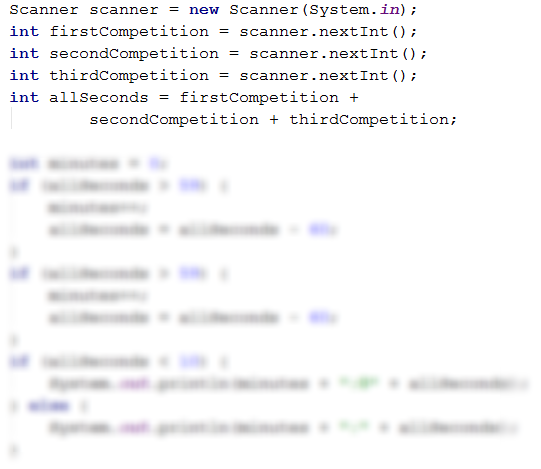A different solution that does not use if-else statement is more appropriate because you can use it for greater time values: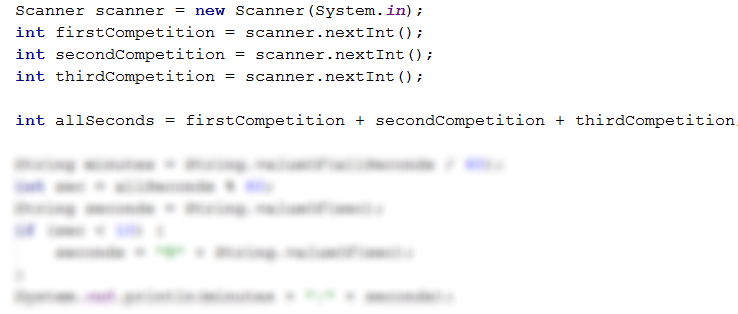### Exercise: Unit converter

Write a program that converts the distance between the following units: m, mm, cm, mi, in, km, ft, yd. Use the conversion table below to convert a value from one unit to another:

Input Unit Output Unit
1 meter (m) 1000 millimeters (mm)
1 meter (m) 100 centimeters (cm)
1 meter (m) 0.000621371192 miles (mi)
1 meter (m) 39.3700787 inches (in)
1 meter (m) 0.001 kilometers (km)
1 meter (m) 3.2808399 feet (ft)
1 meter (m) 1.0936133 yards (yd)

The program will receive three input lines:

• First line: a number for converting.
• Second line: input unit.
• Third line: output unit (for the result).

Input Output
12
km
ft
39370.0788
150
mi
in
9503999.99393599
450
yd
km
0.41147999937455

#### Hint and guidelines

Read the input data. We can use the toLowerCase() function, which will make all letters lowercase. As we can see from the table in the task, we can easily convert values if we use meters as our base unit. Afterwards calculate the result from the conversion of the input number in meters, performing a set of checks to define the input unit. Then calculate directly the output unit.Keep in mind that in Java, you cannot use operator == for string comparison. For this purpose, you may use the built-in functions.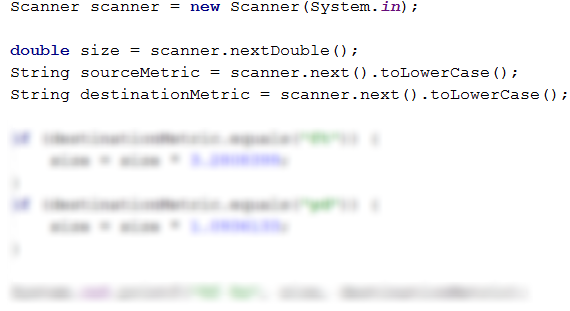## Debugging

There were probably errors in the code you wrote so far. There is an easier way to find errors, use a tool. In the following section, we will look at one.

### What is "Debugging"?

Debugging lets you find and resolve errors, called bugs, a lot faster. Debugging is the process that lets you track step by step the execution of a program This tracking is possible by pausing the execution of the program and analyzing its state by thorough examination, line by line. Of the logic that follows, the defined variables and how they are changed, and so on.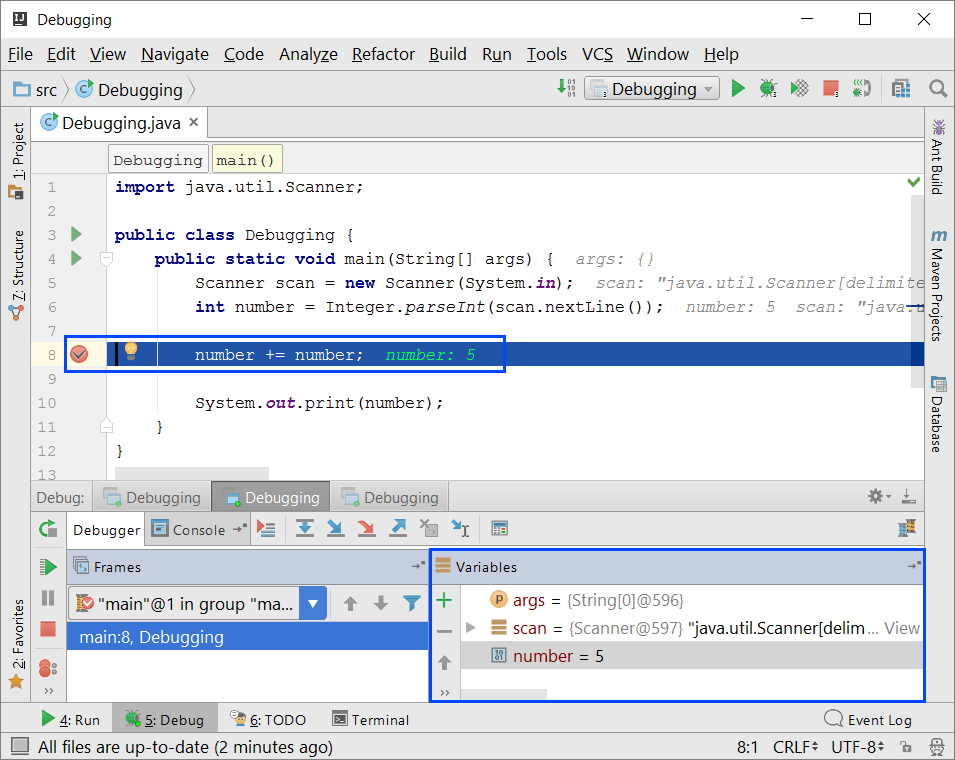### Debugging with IntelliJ Idea

By pressing a combination of buttons [Shift + F9] you run the current program in debug mode. To move to the next line in the code, use the [F7] button.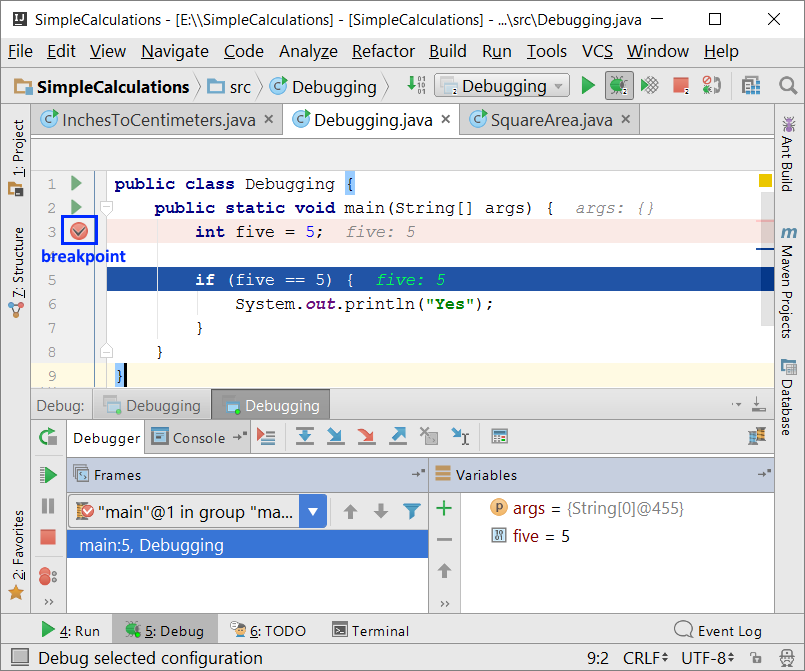By pressing a combination of buttons [CTRL + F8] you create special markers called breakpoints which suspend program execution at a specific point.

## Exercises: Simple conditions

To get a better understanding of what we have learned, let's solve a few practical exercises.

### Empty project in IntelliJ Idea

Create a new project with name Java in IntelliJ Idea and leave all other options at their default state. In order to better organize the solutions of the tasks from the exercises - each solution will be in a separate class and all classes will be in the src directory of the project.

Run IntelliJ Idea. Create a new Java project: [File] → [New] → [Project].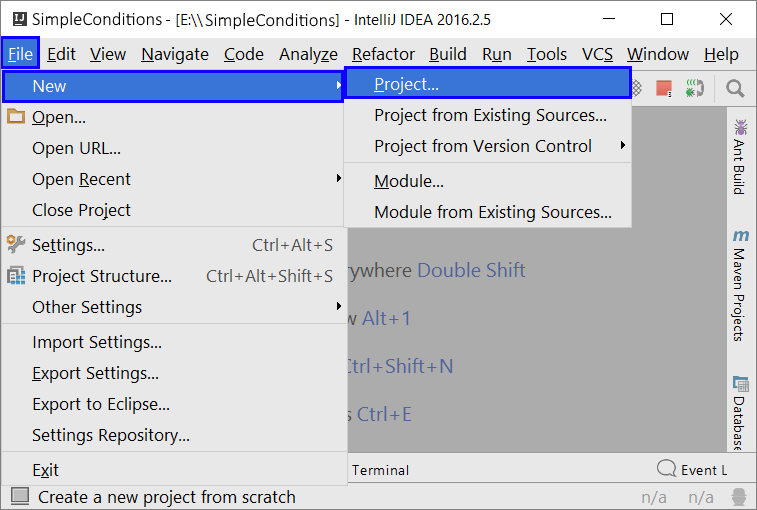Choose Java from the left panel and leave anything else at its default state, press [Next]. In the next dialog box, we have an option to create a project from a template. Usually, we will do this, but now we can skip it and just press [Next]. In the last dialog box, enter the project's name and storage location, and then click [Finish].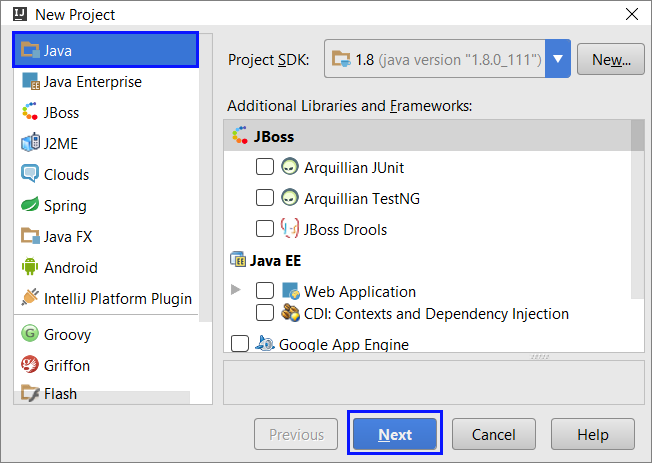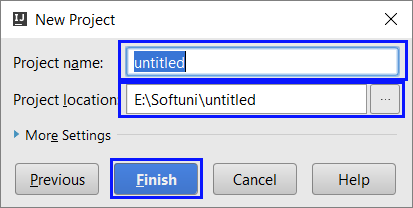We now have an empty Java project: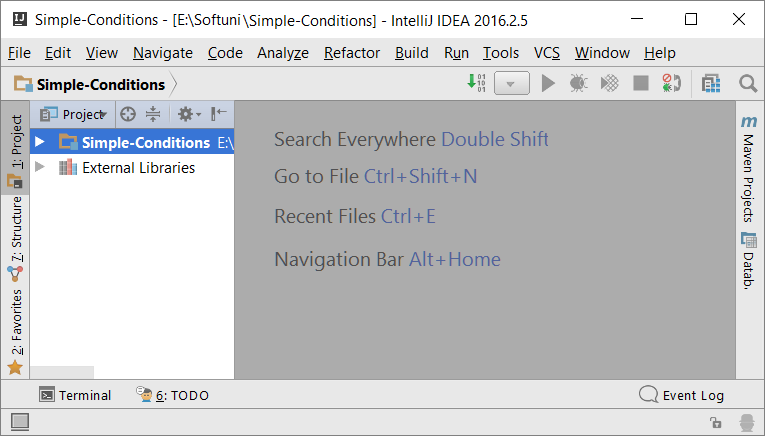### Exercise: Check for excellent grade

The first task of this exercises is to write a program that reads input data from the console. As input data enter a score (decimal number) and output "Excellent!" if the score is 5.50 or above.

Input Output
6 Excellent!
5 (no output)
5.5 Excellent!
5.49 (no output)

#### Hint and guidelines

Create a new class in the existing project in IntelliJ Idea by right-clicking over [src] folder. Choose [New] → [Java Class].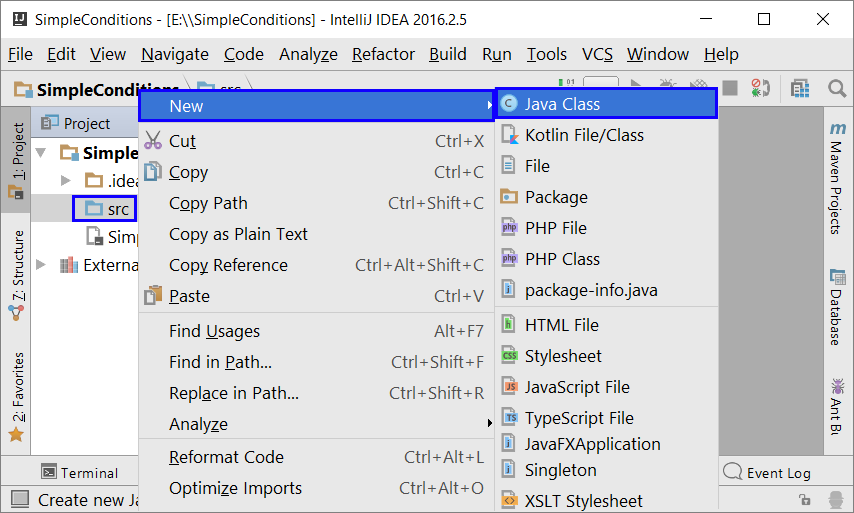A dialog box with two fields will open. In the upper one - enter the name of the class, and in the lower one - the type (there are other options besides classes, but so far they are out of our scope), then press [OK] button to create our class. We set a name, for example, "Excellent-Result":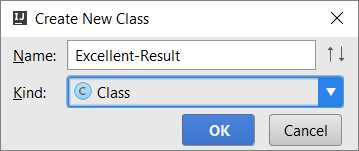We already have a class containing one console application. What's left is to write the code that solves the task.

To do that, go to the body of the method main (string [] args) and place the cursor between the opening and closing curly brackets of the method. If the main method is not created automatically in IntelliJ Idea, there is a keyboard shortcut to do this - psvm. Inside the main method, we write the following code: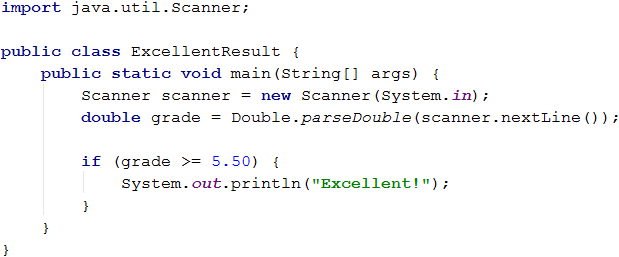Run the program by clicking on the green arrow in front of the class name and test it with different input values: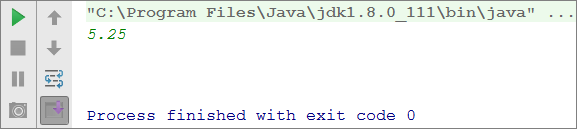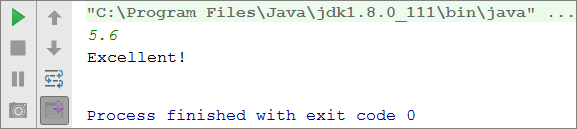#### Test the code in the Judge system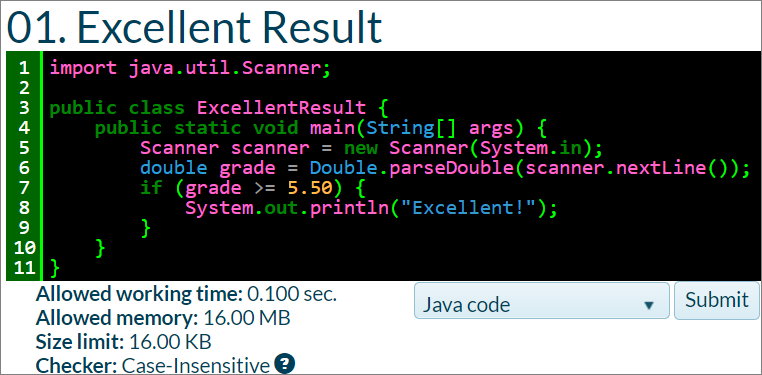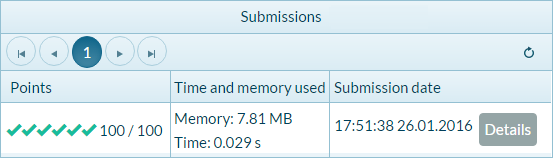### Exercise: Excellent grade or not

The next task of this exercise is to write a program that reads input data from the console. As input data enter a score (decimal number) and output "Excellent!" if the score is 5.50 or above, otherwise "Not excellent!".

#### Sample input and output

Input Output
6 Excellent!
5 Not excellent.
5.5 Excellent!
5.49 Not excellent.

#### Hint and guidelines

Create a new class in the existing project in IntelliJ Idea by right-clicking over [src] folder. Choose [New] → [Java Class].

We already have a class containing one console application. What's left is to write the code that solves the task. We can help ourselves with the sample code from this picture:Run the program by clicking on the green arrow in front of the class name and test it with different input values: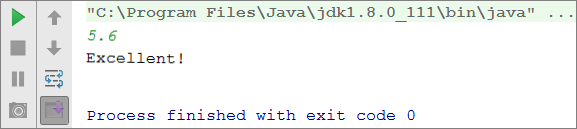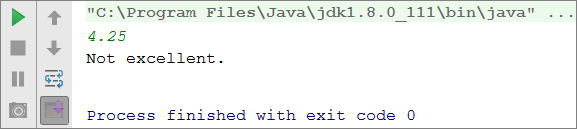#### Test the code in the Judge system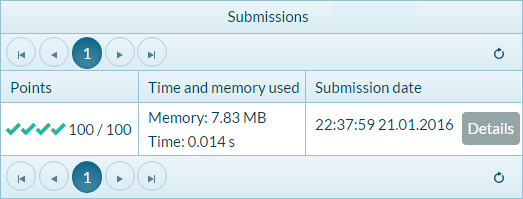### Exercise: Even or odd number

The next task is to write a program that reads input data from the console. As input data enter an integer and output even or odd.

Input Output
2 even
3 odd
25 odd
1024 even

#### Hint and guidelines

Create a new class in the existing project in IntelliJ Idea by right-clicking over [src] folder. Choose [New] → [Java Class].

In the main method, public static void main() write the code to solve the task. To check if a number is even, we can use operator %, which returns the remainder of integer division by 2 in the following way: boolean isEven = (number %2 == 0);.

Run the program by clicking on the green arrow in front of the class name and test it with different input values:### Exercise: Find the greater number

The next task is to write a program that reads input data from the console. As input data enter two integers each on a separate line and output the greater one.

Input Output
5
3
5
3
5
5
10
10
10
-5
5
5

#### Hint and guidelines

Create a new class in the existing project in IntelliJ Idea by right-clicking over [src] folder. Choose [New] → [Java Class]. To solve the task it's necessary to use one if-else statement. You can use the code from the image below. Have in mind that it's blurred on purpose.Run the program by clicking on the green arrow in front of the class name and test it with different input values:### Exercise: Write the number (from 0 to 9 ) in English.

The next task is to write a program that reads input data from the console. As input data, enter an integer in the range [0 … 9] and output the integer in English. If the integer is over the given range, print "number too big".

#### Sample input and output

Input Output
5 five
1 one
9 nine
10 number too big

#### Hint and guidelines

To solve the task, we can use sequential if-else statements to cover all eleven possible cases.

#### Test the code in the Judge system

The next task is to write a program that reads input data from the console. As input data, enter a password (a single line with random text) and check if the input data is the same as the phrase "s3cr3t!P@ssw0rd". Output "Welcome" if the result is true and "Wrong password!" otherwise.

#### Sample input and output

Input Output
s3cr3t!P@ssw0rd Welcome

#### Hint and guidelines

To solve the task it is necessary to use one if-else statement.

### Exercise: Number from 100 to 200

The next task is to write a program that reads input data from the console. As input data, enter an integer and check if the input data is below 100, between 100 and 200 or above 200. Output the corresponding message as shown in the table below.

#### Sample input and output

Input Output
95 Less than 100
120 Between 100 and 200
210 Greater than 200

### Exercise: The same words

The next task is to write a program that reads input data from the console. As input data, enter two words and check if they are the same. Do not distinguish between uppercase and lowercase letters. Output "yes" or "no".

Input Output
Hello
Hello
yes
SoftUni
softuni
yes
Soft
Uni
no
beer
vodka
no
HeLlO
hELLo
yes

#### Hint and guidelines

Before comparing words, transform them to lowercase so that the size of the letters (uppercase/lowercase) doesn't affect the comparison: String wordFirst = scanner.next().toLowerCase().

### Exercise: Speed information

The next task is to write a program that reads input data from the console. As input data, enter speed(a decimal number). Output information about the speed.

• At speed up to 10 (inclusive), print "slowly".
• At speed above 10 and up to 50, print "average".
• At speed above 50 and up to 150, print "fast".
• At speed above 150 and up to 1000, print "ultra fast".
• At greater speed, print "extremely fast".

#### Sample input and output

Input Output
8 slow
49.5 average
126 fast
160 ultra fast
3500 extremely fast

### Exercise: Faces of figures

The next task is to write a program that reads input data from the console. As input data, enter the dimensions of a geometric figure and calculates its face. The figures are of four types: square, rectangle, circle, and triangle.

On the first line of input data, read the type of figure (square, rectangle, circle, triangle).

• If the figure is a square, on the next line read one number - the length of its side.
• If the figure is a rectangle, on the next two lines read two numbers - the lengths of its sides.
• If the figure is a circle, on the next line read one number - the radius of the circle.
• If the figure is a triangle, on the next two lines read two numbers - the length of its side and the length of the adjacent height.

Format the output to 3 digits after the decimal point.

Input Output
square
5
25
rectangle
7
2.5
17.5
circle
6
113.097
triangle
4.5
20
45

### Exercise: Calculate what is going to be the time in 15 minutes

The next task is to write a program that reads input data from the console. As input data, enter the hour and minutes (each on a separate line) of a 24-hour day and calculates what is going to be the time in 15 minutes. Print the output in hh: mm format. The hours are always between 0 and 23, and the minutes are always between 0 and 59. Ourput the hours either using one or two digits. Minutes are always displayed with two digits and a leading zero when necessary.

Input Output
1
46
2:01
0
01
0:16
23
59
0:14
11
08
11:23
12
49
13:04

#### Hint and guidelines

To solve the task, add 15 minutes and perform a few checks. If the minutes exceed 59, increase the hours by 1 and decrease the minutes by 60. Similarly, consider the case when the hours exceed 23. When printing the minutes, check for leading zero.

### Exercise: Equals three numbers

The next task is to write a program that reads input data from the console. As input data, enter 3 integers. Output if they are equal (yes/no).

Input Output
5
5
5
yes
5
4
5
no
1
2
3
no

### Exercise: Write a number from 0 to 100 in English

The next task is to write a program that reads input data from the console. As input data, enter a number in the range [0 … 100] and convert the number into a text. Output the text in English.

Input Output
25 twenty five
42 forty two
6 six

#### Hint and guidelines

To solve the task, first, check for one-digit numbers, and if the number has only one digit, print the appropriate text for it. Then check for two-digit numbers. Print them in two parts: left part (tens = number / 10) and right part (units = number % 10). If the number has three digits, it must be 100 and considered a special case.